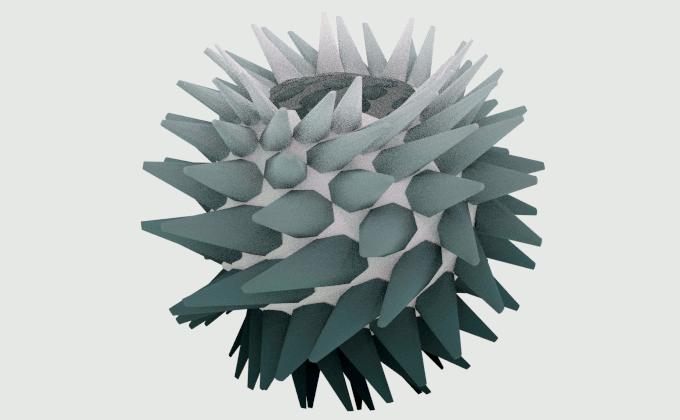# Bloom Spheres in Sverchok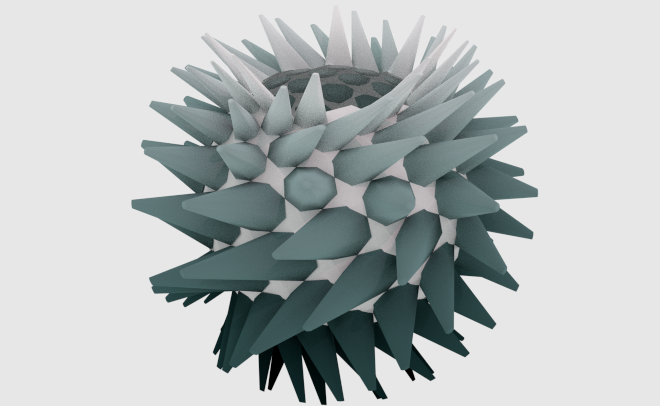These videos by John Edmark of what he calls bloom spheres inspired me to try something similar in Sverchok. Edmark has designed and 3D printed forms that when spun under a strobe light appear to move and twist.

Its possible to produce computer animations that work in the same way. Mangakid has already made some animations in Blender based on Edmark's work. Python code snippets for mangakid's animations can be found on this stackoverflow question and answer.

The first stage to work on bloom spheres in Sverchok was to write a scripted node to produce the basic mesh structure that underlies the blooms. Edmark describes this as:

First a set of points are placed, one-at-a-time, in a cylindrical arrangement. Each point is placed 137.5º around the cylinder's axis from the previous point and also raised a bit...

The next step is to project each point onto the sphere's surface by projecting the point toward the center point of the sphere...

The points are connected with lines to form a quadrilateral mesh.

This is best worked out in spherical coordinates.

The golden angle $$g$$ is given by

\begin{align*} g=\pi (3-\sqrt{5}) &= 2.399963... \textrm{ radians}\\ &= 137.507...^{\circ} \end{align*}

The radius of the sphere is $$r_0$$ and $$z_h$$ is the increment by which the "point is raised a bit". Then for $$i = 0, 1 ... n$$ the points on the bloom sphere are given by:

\begin{align*} \theta (i) &= \frac{i}{g}\\ \phi (i) &= \tan^{-1} \frac{r_0}{iz_h}\\ r(i) &= r_0 \end{align*}

I've coded this up into a scripted node. To use the bloom sphere node in Blender first install the Sverchok addon. Download the bloom sphere code from github. Then load the python file as a text blocks into a blend file. Add a Scripted Node to a Sverchok node tree. On the node select the bloom_sphere.py code from the lower drop down. Then click the plugin icon to the right of this field. The node should turn blue with some inputs and outputs. Wire the Verts and Faces outputs to a Viewer Draw node and you should see some geometry.

It is also possible to use the XYZ function surface that is part of the Extra Objects addon for Blender to generate the mesh vertices. I found it much harder to get the addon to join then up to form the correct quadrilateral mesh. It was much easier to do this in my Sverchok scripted node.

The Frame Info node can be easily used to rotate the bloom sphere 137.5° every frame. Set the end frame to 145 for a continuous loop animation.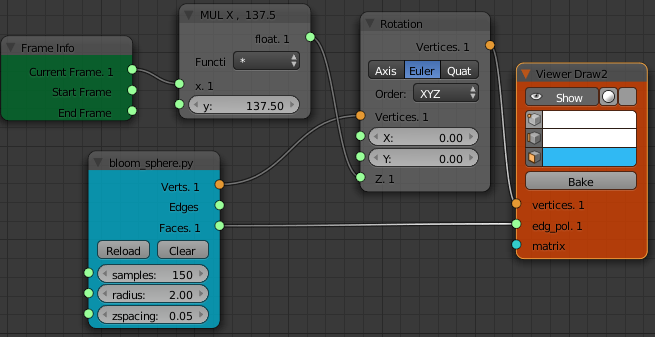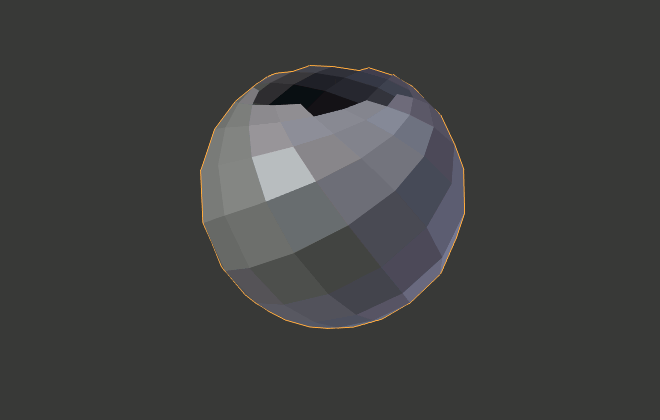The same node diagram can also be used to animate any of John Edmark's stl files that he has made available here under a Creative Commons BY NC SA license. Just import the stl into Blender and replace the Scripted Node with a Object Scene node.

Alternatively to spin a mesh outside Sverchok, make a key frame animation where the first frame has no rotation and the End frame has 137.5° * (number of frames - 1) rotation about the z-axis.

For example:

• Select the object to be rotated
• Set the End Frame to 145
• Change the Active Keying Set to "Rotation"
• Insert a Key Frame on the first frame
• Move to the last frame
• Set the rotation of the object around the z-axis to 19800 degrees (144 * 137.5° = 19800)
• Insert a Key Frame on this frame
• Set the Current Frame back to 1
• Open up a Graph Editor view
• Change the Interpolation Mode for the animation curve to linear. Using the menu: Key > Interpolation Mode > Linear or using the keyboard T L.
• Check that stepping along one frame changes the Rotation Z angle by 137.5°

This will give a seamless 6 second (145 frames/ 24 frames per second ~= 6 seconds) animation. It is seamless because 19800 is a whole multiple of 360 (360° * 55 = 19800°).

Note: Any rotation angle where

$$g_{n,m} = ng - m2\pi$$

where $$n= 1,2,..$$ anf $$m$$ is an integer chosen to map the angle back to the interval $$-\pi$$ to $$\pi$$ will also work. For example rotating any of 137.5°, -85°, 52.5°, 170°, -32.5°, 105°, -117.5°, or 20° per frame will work but for each angle the animation will appear to have a different speed.

Now comes the fun part, editing the mesh to produce interesting animations. John Edmark describes:

Each of the quadrilaterals is populated with an appendage ...

We could do this following my Centers Polygon example but I'll use the Adaptive Polygons node to show another way for a different look.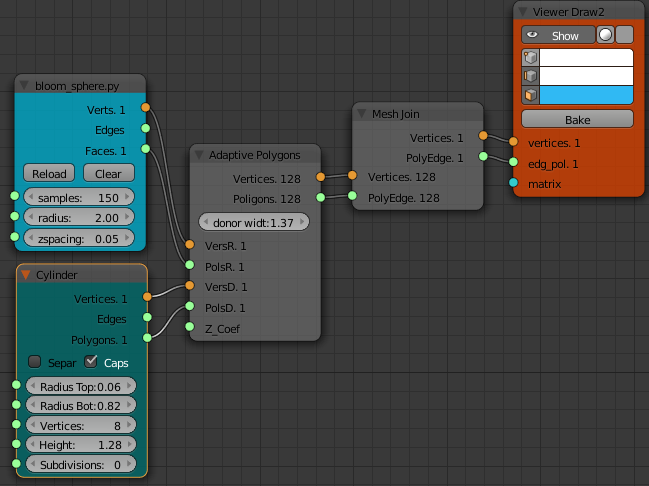I've removed the nodes used to achieve the rotation to simplify the above node diagram.John Edmark then goes on to describe:

In order to make the appendages appear to move back and forth when animated, their tips are sequentially distorted side-to-side (in a sinusoidal motion) as they are placed.

The simplest way of doing something similar to this is to move each vertex side to side before the Adaptive Polygons node. Adding "Side to side" motion is most easily thought of in spherical coordinates. If we vary the "phi" polar coordinate of a point it will move side to side.

Sverchok now has nodes to change the x, y, z coordinates of a vertex into polar coordinates and change them back again. Here we apply a sinusoidal offset to each vertex. The frequency of the sinusoid is varied by changing the stop value of the Float Series node. A multiple (or fraction) of $$\pi$$ is sensible. A Float entry node is used to change the amplitude of the sinusoid.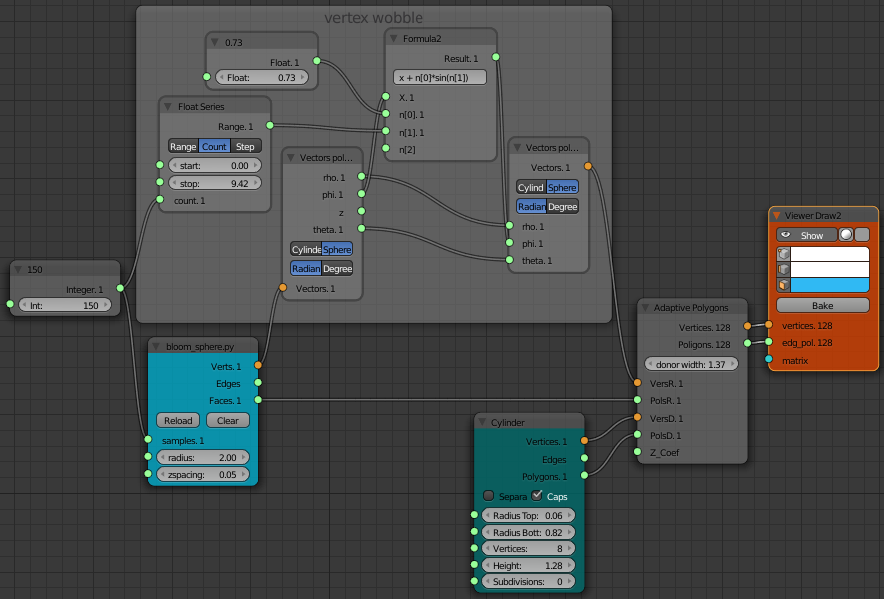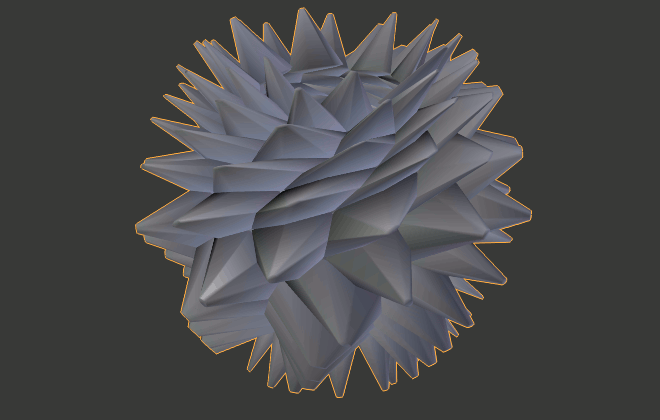With a bit more thought (and a few more nodes!) we can actually wobble just the ends of our appendages adaptive polygons.

First we need to separate out the end vertices with a List Mask (out) node. For the mask we use the length of each vertex (with a Vector Math node) to select those vertices not on the surface of the bloom sphere. It takes a little messing around with List Length nodes to get the range of numbers to use as the angle input for the sinusoid. This multi-level list needs to have the same angle value for all the points at the end of single spike and then increment for the next spike.

The two sets of vertices (the end vertices with the sinusoidal wobble and the original vertices on the sphere) are put back together with a List Mask Join (in) node using the same mask we used for separating them.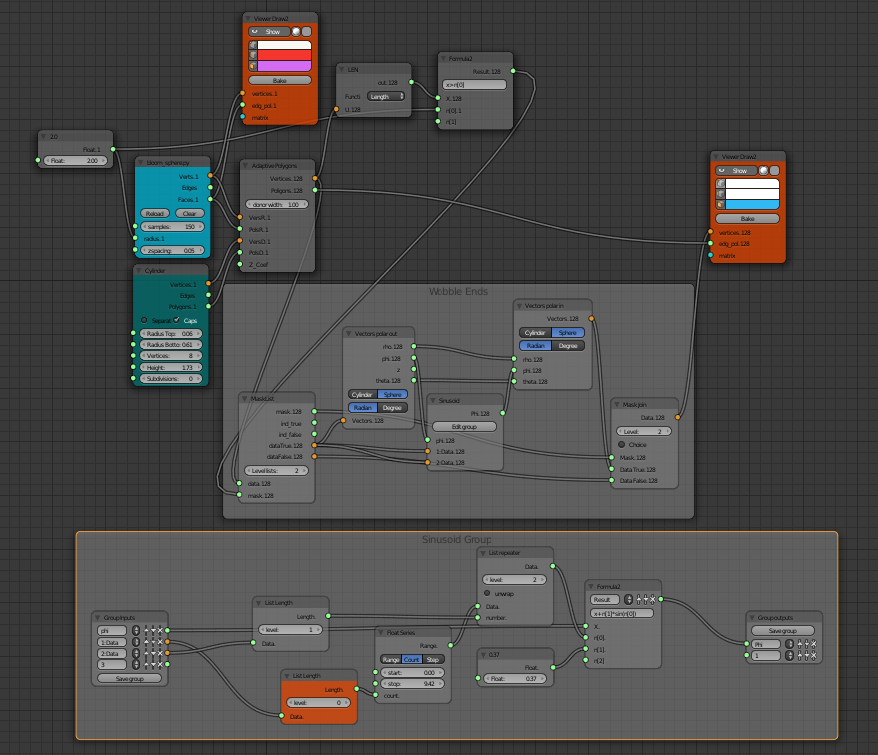to get an animation like this: